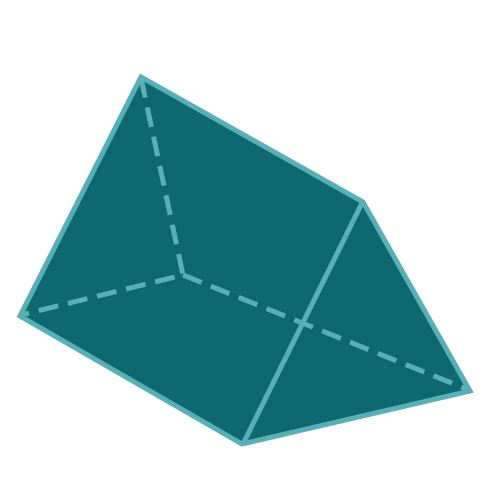Report a Problem
Suggestions

#Calculate The Surface Area of A Triangular Prism

Last updated: Saturday, April 29, 2023
Select a type of prism below
Triangular Prism
Equilateral Triangular Prism
Rectangular Prism
Square Prism
Pentagonal Prism
Hexagonal Prism

The surface area of a triangular prism is a key concept in geometry that pertains to the total area covering the external faces of the three-dimensional shape. A triangular prism consists of two congruent triangles at the ends, known as bases, connected by three parallelogram-shaped lateral faces. The concept of surface area has broad applications in various fields, including engineering, architecture, and design, where it is crucial to estimate material requirements, costs, and structural integrity.

Triangular prisms, like other three-dimensional shapes, have numerous real-life applications that make understanding their surface area essential. For instance, in construction and architecture, the surface area plays a role in determining the stability and strength of structures, as well as insulation and energy efficiency. In packaging design, calculating the surface area of a triangular prism helps optimize material usage, reduce waste, and minimize costs. Moreover, artists and designers frequently employ triangular prisms in their creations, making the knowledge of surface area invaluable for conceptualizing and executing their work.

While there is no definitive historical account of the origin of the triangular prism or its surface area concept, it can be traced back to ancient Greece, where mathematicians like Euclid and Pythagoras laid the groundwork for modern geometry. Their studies on triangles, parallelograms, and three-dimensional shapes have greatly influenced contemporary understanding of geometry and the surface area of various shapes, including triangular prisms. Today, the surface area of a triangular prism remains a fundamental principle in geometry and continues to serve as a crucial element in a multitude of practical applications.

The formula for determining the surface area of a triangular prism is defined as:
$$where$$
$$s$$ $$=$$ $$\dfrac{a + b + c}{2}$$
$$SA$$: the surface area of the prism
$$a$$: the length of side a of the triangular base
$$b$$: the length of side b of the triangular base
$$c$$: the length of side c of the triangular base
$$d$$: the depth of the prism
The SI unit of surface area is: $$square \text{ } meter\text{ }(m^2)$$

## Find $$SA$$

Use this calculator to determine the surface area of a triangular prism when all sides are given.
Hold & Drag
CLOSE
the length of side a of the triangular base
$$a$$
$$meter$$
the length of side b of the triangular base
$$b$$
$$meter$$
the length of side c of the triangular base
$$c$$
$$meter$$
the depth of the prism
$$d$$
$$meter$$
Bookmark this page or risk going on a digital treasure hunt again